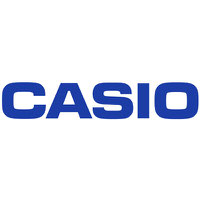# Casio, FX-55PLUS, True Fraction Display

 MFG #: FX-55PLUS ATECH #: CASFX55PLUS UPC : 079767900854 Manufacturer : CASIO

### Marketing Information

With Natural Textbook Display™, the fx-55 PLUS introduces learners to fractions, division-with-remainder, and random numbers. Natural Textbook Display presents mixed numbers and improper fractions exactly as they appear on paper, and helps students understand the fundamentals of fractional simplification. The fx-55 PLUS expresses the joy of math through a colorful presentation of functions on the keypad.

### Technical Specifications

• Product features: Non Programmable; Non Graphing
• Product type: Non Programmable; Non Graphing
• Number of digits: 10 + 2 digits
• Liquid crystal display: Natural textbook display; Dot matrix display
• Key characteristics: Plastic keys
• Key functions: Negative Sign; Power Off
• Memory: No. of memory: 1 ; Independent Memory (M+ / M- / STO M / RCL M); Answer Memory; Memory Protection; Reset Function
• Power supply: Two way Power; Approximate battery life Main 3 years; Auto Power Off
• Accessories: Hard Case
• Display format settings: Number Format: Fix; Number Format: Norm; Toggling Calculation Results (Fraction)
• Calculation Functions: Basic calculation; Basic Calculation; Bracket Calculation; Degree, Minute, Second (Sexagesimal) Calculations; Reciprocal Calculation; Percent Calculation (scientific calculator function); Pi; Irrational number calculation; Rounding Function (Rnd); Rounding Function
• Applied calculation: Continuous Calculation; Replay Function; Multi-replay Function; Remainder Calculation
• Basic functions: Basic Mathematical Functions; Number of Functions : 240; Power Function (Square); Power Function; Exponential Calculation; Simplify
 Net Weight: 0.08 lbs USPS OK UOM: EACH Product Type: Calculator Warranty: 1 Year Country of Origin: CN

### Recent Invoices (showing the last 15 invoices)

Invoice No PO Number Ship to Name Quantity Unit Price Date